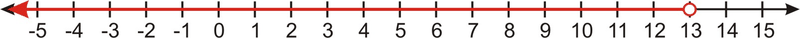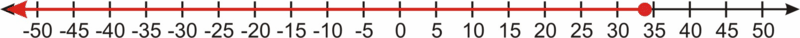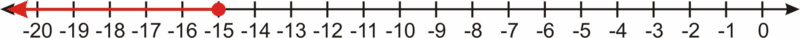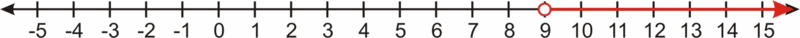# 3.1: Solve One Step Linear Inequalities

•• Contributed by CK12
• CK12

## Linear Inequalities

Could you solve an inequality like x−12>−5?

### Linear Inequalities

To solve an inequality we must isolate the variable on one side of the inequality sign. To isolate the variable, we use the same basic techniques used in solving equations.

We can solve some inequalities by adding or subtracting a constant from one side of the inequality.

#### Solve the inequality and graph the solution set.

x−3<10

Starting inequality: x−3<10

Add 3 to both sides of the inequality: x−3+3<10+3

Simplify: x<13#### Solve the inequality and graph the solution set.

x−20≤14

Starting inequality: x−20≤14

Add 20 to both sides of the inequality: x−20+20≤14+20

Simplify: x≤34Solving Inequalities Using Multiplication and Division

We can also solve inequalities by multiplying or dividing both sides by a constant. For example, to solve the inequality 5x<3, we would divide both sides by 5 to get x<35.

However, something different happens when we multiply or divide by a negative number. We know, for example, that 5 is greater than 3. But if we multiply both sides of the inequality 5>3 by -2, we get −10>−6. And we know that’s not true; -10 is less than -6.

This happens whenever we multiply or divide an inequality by a negative number, and so we have to flip the sign around to make the inequality true. For example, to multiply 2<4 by -3, first we multiply the 2 and the 4 each by -3, and then we change the < sign to a > sign, so we end up with −6>−12.

The same principle applies when the inequality contains variables.

#### Solve the inequality.

4x<24

Original problem: 4x<24

Divide both sides by 4: 4x4<244

Simplify: x<6

#### Solve the inequality.

−5x≤21

Original problem: −5x≤21

Divide both sides by -5 : −5x−5≥21−5 Flip the inequality sign.

Simplify: x≥−215

### Examples

Example 3.1.1

Solve the inequality.

x+8≤−7

Solution

Starting inequality: x+8≤−7

Subtract 8 from both sides of the inequality: x+8−8≤−7−8

Simplify: x≤−15Example 3.1.2

x+4>13

Solution

Starting inequality: x+4>13

Subtract 4 from both sides of the inequality: x+4−4>13−4

Simplify: x>9Example 3.1.3

x/25<3/2

Solution

Original problem: x/25<3/2

Multiply both sides by 25: 25⋅(x/25)<(3/2)⋅25

Simplify: x<75/2 or x<37.5

Example 3.1.4

x/−7≥9

Solution

Original problem: x−7≥9

Multiply both sides by -7: −7⋅(x/−7)≤9⋅(−7) Flip the inequality sign.

Simplify: x≤−63

### Review

For 1-8, solve each inequality and graph the solution on the number line.

1. x−5<35
2. x+15≥−60
3. x−2≤1
4. x−8>−20
5. x+11>13
6. x+65<100
7. x−32≤0
8. x+68≥75

For 9-12, solve each inequality. Write the solution as an inequality and graph it.

1. 3x≤6
2. x/5>−3/10
3. −10x>250
4. x/−7≥−5

### Vocabulary

Term Definition
distributive property The distributive property states that the product of an expression and a sum is equal to the sum of the products of the expression and each term in the sum. For example, a(b+c)=ab+ac.
Linear Inequality Linear inequalities are inequalities that can be written in one of the following four forms: ax+b>c,ax+b<c,ax+b≥c, or ax+b≤c.